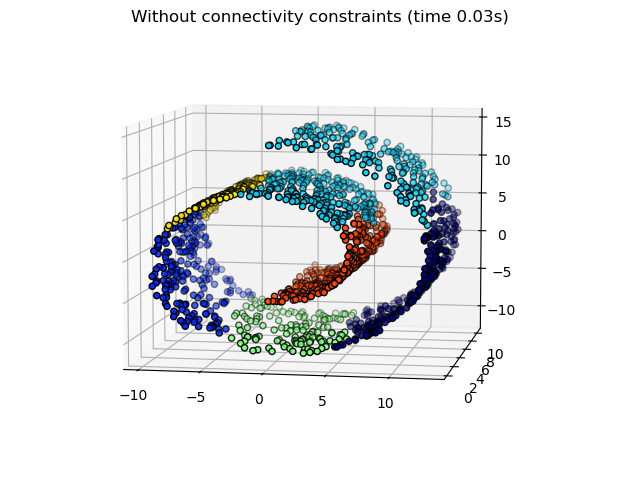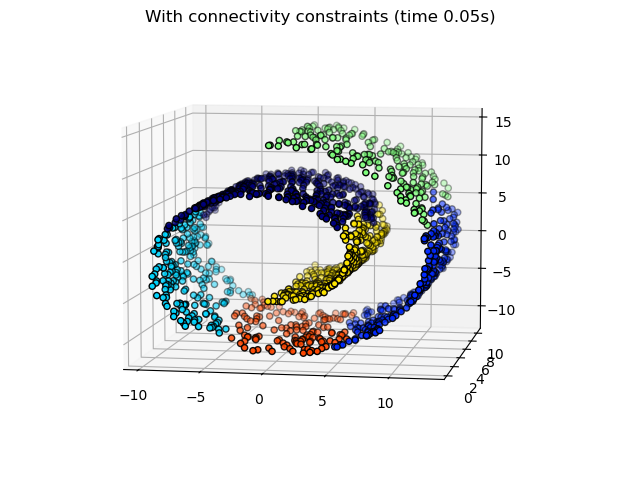# Hierarchical clustering: structured vs unstructured ward¶

Example builds a swiss roll dataset and runs hierarchical clustering on their position.

In a first step, the hierarchical clustering is performed without connectivity constraints on the structure and is solely based on distance, whereas in a second step the clustering is restricted to the k-Nearest Neighbors graph: it’s a hierarchical clustering with structure prior.

Some of the clusters learned without connectivity constraints do not respect the structure of the swiss roll and extend across different folds of the manifolds. On the opposite, when opposing connectivity constraints, the clusters form a nice parcellation of the swiss roll.

# Authors : Vincent Michel, 2010
#           Alexandre Gramfort, 2010
#           Gael Varoquaux, 2010

import time as time

# The following import is required
# for 3D projection to work with matplotlib < 3.2
import mpl_toolkits.mplot3d  # noqa: F401
import numpy as np


## Generate data¶

We start by generating the Swiss Roll dataset.

from sklearn.datasets import make_swiss_roll

n_samples = 1500
noise = 0.05
X, _ = make_swiss_roll(n_samples, noise=noise)
# Make it thinner
X[:, 1] *= 0.5


## Compute clustering¶

We perform AgglomerativeClustering which comes under Hierarchical Clustering without any connectivity constraints.

from sklearn.cluster import AgglomerativeClustering

print("Compute unstructured hierarchical clustering...")
st = time.time()
elapsed_time = time.time() - st
label = ward.labels_
print(f"Elapsed time: {elapsed_time:.2f}s")
print(f"Number of points: {label.size}")

Compute unstructured hierarchical clustering...
Elapsed time: 0.03s
Number of points: 1500


## Plot result¶

Plotting the unstructured hierarchical clusters.

import matplotlib.pyplot as plt

fig1 = plt.figure()
ax1 = fig1.add_subplot(111, projection="3d", elev=7, azim=-80)
ax1.set_position([0, 0, 0.95, 1])
for l in np.unique(label):
ax1.scatter(
X[label == l, 0],
X[label == l, 1],
X[label == l, 2],
color=plt.cm.jet(float(l) / np.max(label + 1)),
s=20,
edgecolor="k",
)
_ = fig1.suptitle(f"Without connectivity constraints (time {elapsed_time:.2f}s)")## We are defining k-Nearest Neighbors with 10 neighbors¶

from sklearn.neighbors import kneighbors_graph

connectivity = kneighbors_graph(X, n_neighbors=10, include_self=False)


## Compute clustering¶

We perform AgglomerativeClustering again with connectivity constraints.

print("Compute structured hierarchical clustering...")
st = time.time()
ward = AgglomerativeClustering(
).fit(X)
elapsed_time = time.time() - st
label = ward.labels_
print(f"Elapsed time: {elapsed_time:.2f}s")
print(f"Number of points: {label.size}")

Compute structured hierarchical clustering...
Elapsed time: 0.05s
Number of points: 1500


## Plot result¶

Plotting the structured hierarchical clusters.

fig2 = plt.figure()
ax2 = fig2.add_subplot(121, projection="3d", elev=7, azim=-80)
ax2.set_position([0, 0, 0.95, 1])
for l in np.unique(label):
ax2.scatter(
X[label == l, 0],
X[label == l, 1],
X[label == l, 2],
color=plt.cm.jet(float(l) / np.max(label + 1)),
s=20,
edgecolor="k",
)
fig2.suptitle(f"With connectivity constraints (time {elapsed_time:.2f}s)")

plt.show()Total running time of the script: (0 minutes 0.333 seconds)

Gallery generated by Sphinx-Gallery